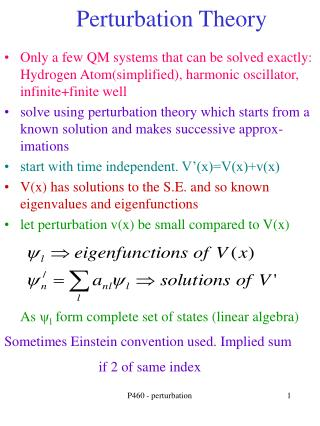DownloadDownload PresentationPerturbation Theory

# Perturbation Theory

Download Presentation## Perturbation Theory

- - - - - - - - - - - - - - - - - - - - - - - - - - - E N D - - - - - - - - - - - - - - - - - - - - - - - - - - -
##### Presentation Transcript

1. Perturbation Theory • Only a few QM systems that can be solved exactly: Hydrogen Atom(simplified), harmonic oscillator, infinite+finite well • solve using perturbation theory which starts from a known solution and makes successive approx- imations • start with time independent. V’(x)=V(x)+v(x) • V(x) has solutions to the S.E. and so known eigenvalues and eigenfunctions • let perturbation v(x) be small compared to V(x) As yl form complete set of states (linear algebra) Sometimes Einstein convention used. Implied sum if 2 of same index P460 - perturbation

2. Plug into Schrod. Eq. • know solutions for V • use orthogonality • multiply each side by wave function* and integrate • matrix element of potential v is defined: P460 - perturbation

3. One solution: assume perturbed wave function very close to unperturbed (matrix is unitary as “size” of wavefunction doesn’t change) • assume last term small. Take m=n. Energy difference is expectation value of perturbing potential **** P460 - perturbation

4. Redo compact notation • eigenvalues/functions for a “base” Hamiltonian • want to solve (for l small)(l keeps track or order) • define matrix element for Hamiltonian H • finite or infinite dimensional matrix. If finite (say 3x3) can use diagonalization techniques. If infinite can use perturbation theory • write wavefunction in terms of eigenfunctions but assume just small change P460 - perturbation

5. compact notation- energy • look at first few terms (book does more) • which simplifies to (first order in l) • rearranging • take the scalar product of both sides with • first approximation of the energy shift is the expectation value of the perturbing potential P460 - perturbation

6. compact notation- wavefunction • look at wavefunction • and repeat equation for energy • take the scalar product of both sides with • gives for first order in l • note depends on overlap of wavefunctions and energy difference P460 - perturbation

7. Time independent example • know eigenfunctions/values of infinite well. • Assume mostly in ground state n=1 P460 - perturbation

8. Time independent example • Get first order correction to wavefunction • only even Parity terms remain (rest identically 0) as • gives Even Parity P460 - perturbation